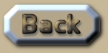Shape of the Universe

 Shape of the Universe The Universe has an unusual shape to it. To understand this shape, we have to consider ancient people trying to comprehend the Earth being spherical. For them, the Earth appeared flat. But the real shape of the Earth could be better described as a flat 2d-surface on top of a 3d-sphere. By the same token our Universe is a 3d-hyper-surface, on top of a 4d-hyper-sphere. Now where Sum Theory differs from Relativity, is that time is not considered a true dimension. Here, time is immaculate – it is not conflated with space in the same way that the Relativists use the term ‘space-time’. So in Sum Theory, the 4th dimension is actually another dimension of space. Time is something else entirely. This 4th dimension of space is not quite the same as the 3 dimensions that we commonly observe. Light and gravity move along our 3d-hyper-surface, and are only very marginally altered by the curvature of the 4th spatial dimension. In this sense, Sum Theory is certainly easier to comprehend than Relativity because there is no bamboozling conflation between space and time like there is in Relativity. So to picture how Sum Theory conceptualizes the shape of the Universe, all we have to do is envision how the Earth appears to be flat, but is in fact a sphere, and then simply add another dimension of space. That is much easier to do mathematically, than it is graphically, because we are very bad at being able to imagine something that we have not clearly observed before. But, like most things, with time and practise – it does get easier. The reason why Sum Theory replaces space-time with 4d-space, is discussed at length in previous chapters. Here, all we need to appreciate is that when astronomers observe that the most distance galaxies are retreating from us at highest velocities – the analogy of the inflating balloon is most apt. So by similar analogy: if the Earth was hypothetically inflating then the further away an object was from the observer, the faster they would appear to be moving away from each other. So no point on the surface is at the ‘centre’. Of course light and gravity would need to bend slightly with the 3d-hyper-surface. In Sum Theory light and gravity are both confined to the 3d-hyper-surface. We know this because light and gravity both obey the inverse of the square law. If light or gravity were transmitted through 4d-space then they would obey the inverse of a cube law. So our 3d-Universe is trapped ‘on top’ of a 4d-shape, much the same as a 2d-surface is fixed ‘on top’ of a sphere. Thus, in order for the Universe to expand, it must be constantly increasing its hyper-volume in truly 4d terms. The big assumption I make is that the amount of ‘4d-stuff’ that is inflating the Universe is doing so at a constant rate. This may prove to not be constant, but the discrepancies in the rate of expansion are so varied that I can see no solid reason to consider it one way or the other. Adjustments may be required when the data becomes better; but for now we’ll just assume it inflates at a constant rate of energy. The 4d-stuff inflating the Universe, is thus actually one aspect of dark energy. It appears as if dark energy consists of two different features – because I have already demonstrated that dark energy is also ‘spin’. This was the only way that uniform orbital structures could appear throughout the Universe: if the mass of the Universe was spinning at an increasing rate at the very beginning. (See previous chapters). The possibility of an open-ended Universe consisting of endless 3d-space, also needs to be considered. This is the obvious observation, much like it is obvious that the ‘Earth is flat’. If we take this as a premise we reach the conclusion that the rest of the Universe is receding from our particular vantage point, making us the centre of the Universe due to the increasing red-shift of galaxies furthest from us. Whilst not illogical, we can safely conclude that this is unlikely for purely inductive reasons. That is why the only alternative has to be that the Universe is a 4d-hyper-sphere – because then the ‘edges’ of the 3d-Universe must wrap around in 4d-space much the same way that a 2d-surface has no edge if it wraps around to form a 3d-sphere. That the Universe is expanding, leads to the apt analogy of an inflating balloon. Now one of the features of an inflating balloon is that if the air is entering at a steady rate, then it appears from the perspective of diameter that the early stages expand most rapidly. And at the later stages, expansion seems to slow down. So it is a matter of perspective as to whether or not the rate of expansion is slowing down, or increasing. The key question being: Are we considering this from the perspective of diameter over time; or from the perspective of volume over time; or energy over time? None of the other theories seem to account for this detail. So there are two vital formulae for calculating the shape of the Universe: .
 You may wish to compare these two formulae to the formula for a 3d-sphere and the formula for the 2d-surface of a 3d-sphere. They may be similar but they are not the same. So at this point we need to consider the next aspect of the size of the Universe: its density. .This is an extract summary of Chapter XXX of the book: Flight Light and Spin